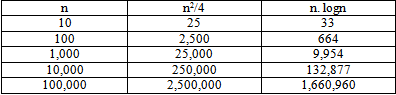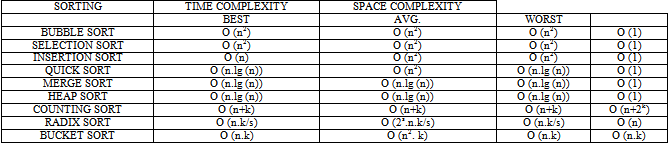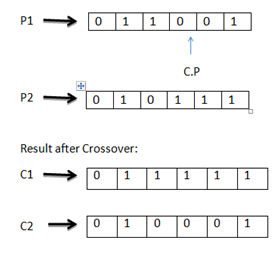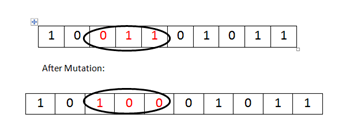﻿ Genetic Algorithm Based on Sorting Techniques

Genetic Algorithm Based on Sorting Techniques

Parul Aggrawal, Faisal Naved, Mohd HaiderOPEN ACCESSPEER-REVIEWED

Genetic Algorithm Based on Sorting Techniques

Parul Aggrawal1,, Faisal Naved1, Mohd Haider1

1Department of Computer Science, Jamia Hamdard University, New Delhi-62, India

Abstract

Genetic Algorithm, an Artificial Intelligence approach is based on the theory of natural selection and evolution. Traditional methods of sorting data are too slow in finding an efficient solution when the input data is too large. In contrast, Genetic Algorithm generates fittest solutions to a problem by exploiting new regions in the search space. This paper targets the three most commonly used Bubble, Selection and Insertion sorting techniques and executes memory on an input ranging from 1,000 to 10,000 where the input is entered in increasing, decreasing and random order. It mainly uses the Genetic Algorithm approach to optimize the effect of the three algorithms by generating an output which is consistent in terms of time variations which is not otherwise. This has been achieved by exploiting the property of Genetic Algorithm by choosing best parameter for population size, encoding, selection criteria, operator choice and optimized fitness function.

At a glance: Figures

1234
Prev Next

• Aggrawal, Parul, Faisal Naved, and Mohd Haider. "Genetic Algorithm Based on Sorting Techniques." Journal of Computer Sciences and Applications 3.2 (2015): 40-45.
• Aggrawal, P. , Naved, F. , & Haider, M. (2015). Genetic Algorithm Based on Sorting Techniques. Journal of Computer Sciences and Applications, 3(2), 40-45.
• Aggrawal, Parul, Faisal Naved, and Mohd Haider. "Genetic Algorithm Based on Sorting Techniques." Journal of Computer Sciences and Applications 3, no. 2 (2015): 40-45.

 Import into BibTeX Import into EndNote Import into RefMan Import into RefWorks

1. Introduction

Section 1 of this paper comprises of introduction to the basic sorting and Genetic Algorithm. Section 2 comprises of related work in which average elapsed time is calculated at different inputs for Bubble, Insertion and Selection Sort. Section 3 contains the Experimental Results in which Inconsistency in Bubble Sort, Insertion sort and Selection sort is shown when inputs are entered in increasing order, decreasing order and randomly. Section 4 is the proposed work containing the Algorithm. Section 5 is the Conclusion and future scope. Section 6 are the references in support to the research paper

Sorting is considered as the most fundamental problem while studying Algorithms. The basic principle behind sorting a sequence of n numbers is that its elements are placed randomly and needs to be reordered in ascending order..Search preprocessing is the most important application of sorting. When the values are in sorted order, a better approach is to use binary search. O (n2) time proportion will be taken by an inefficient algorithm where n is equal to the number of elements in an array, whereas, an efficient algorithm takes O (n. lgn) time proportion to sort sequence of numbers where n is the size of the array.For small inputs, we cannot see a big difference, but if we have a larger input (say Population of a city) we can see that an enormous amount of usability played off between the efficient and inefficient algorithms:

Population of a city: =6 million people

n=6 X 106 steps needed

n2=36 X 1012

=3600 X 1010

=3600 seconds (Assuming that 1010 steps are executed in 1 sec)

n.lgn =106 X 20 X 36 => 1 sec

So, if we have to sort a population of 6 million people, only 1 sec is taken by efficient algorithm.O (n log n) time is needed to sort large data because, sorting by 0 (n2) becomes impossible if we have a large data. There are lots of applications, besides simply looking for the maximum or minimum:-- For finding duplicates in a set: sort first, then duplicates will appear next to each other, and can be found by scanning through the sorted array. In finding similar values. In Histograms (counting frequencies): sort first, then do a single pass: repeated items will occur in bunches, and can be counted easily. Note that there are other ways to this. Intersection: given two arrays, what values do they have in common? Sort both of them, and march down both lists, advancing down the list with the smaller value each time. You will find the common values easily. Setting data up for later fast searching: if your data is sorted, you can use binary search to find values in O (log (n)) time.

Sorting is defined as follow:

Input: A sequence of n numbers < A1, A2, A3……………. An >

Output: A permutation (reordering) < A1’, A2, ’, A3, ’……………. An’ > of input sequence such that A1’ <= A2’ <=……… <= An’. 

There are several sorting algorithms. Some of them are Bubble sort, Selection Sort, Insertion Sort, Quick sort, Merge sort, Heap sort, Counting Sort, Radix sort, Bucket sort. The Time and Space Complexities of these sorting techniques are:1.1. Genetic Algorithm

Genetic Algorithms  are the adaptive heuristic search algorithms which are based on the process of growth and development . The basic concept of genetic algorithm follows the principle of survival of the fittest, which was given by Charles Darwin. In 1960’s , John Holland discovered the genetic algorithm. This is the process of moving the chromosome of one population to a new population with the help of some operators such as selection, crossover and mutation.

A population is comprised of a finite value of chromosomes, each chromosome contains a number of genes .

A gene is represented by a binary number or decimal number according to the problem or just the sake of simplicity, this gene value is sometimes called as an allele.

1.2. Some Genetic Algorithm Operators

SELECTION: - This operator is used to select chromosomes for reproduction. Fitter chromosome has a higher probability of selection.

CROSSOVER: - This operator moves the genes of two parent chromosomes to create two new child's chromosomes .

No. of crossover = (Crossover rate*number of a chromosome * number of genes in a chromosome) /100MUTATION: - Genetic diversity from one population to another is performed by mutation operator. This alters the genes or chromosome of the population.

Steps of Genetic Algorithm: Generate an initial population randomly as you want either in binary or in decimal format. Then the fitness of each chromosome is calculated. Selection operator is applied on the population. Crossover operator is applied on the population. Mutation operator is applied on whole population. These processes are repeated until we get the fittest chromosome or until we get the output.2. Related Work

In our approach, we have taken the three sorting algorithms namely bubble sort, insertion sort and selection sort in increasing order, decreasing order and randomly in order to check the elapsed time taken by each sorting technique. In this section we have shown the elapsed time taken by each sorting technique with the help of tables and charts. Table 1 and the corresponding chart (Figure 1) shows the average elapsed time taken by insertion sort when inputs are entered ranging from 1,000 to 10,000 first in increasing order then in decreasing order and then the inputs are taken randomly.

Table 1. Average Elapsed Time of Insertion sort at different inputs

Table 2 and the corresponding chart (Figure 2) shows the average elapsed time taken by Bubble sort when inputs are entered ranging from 1,000 to 10,000 first in increasing order then in decreasing order and then the inputs are taken randomly.

Table 2. Average Elapsed Time of Bubble sort at different inputs

Similarly, Table 3 and the corresponding chart (Figure 3) shows the average elapsed time taken by selection sort when inputs are entered ranging from 1,000 to 10,000 first in increasing order then in decreasing order and then the inputs are taken randomly.

3. Experimental Results

Each input is entered (ranging from 1,000 to 10,000) five times in order to check the consistency of the following sorting techniques. Table 4, Table 5, Table 6, Table 7, Table 8, Table 9, Table 10, Table 11, Table 12, Table 13 and the corresponding charts (Figure 4, Figure 5, Figure 6, Figure 7, Figure 8, Figure 9, Figure 10, Figure 11, Figure 12, Figure 13) shows the inconsistency in elapsed time for different sorting techniques and each input entered five times consecutively on Windows 7 operating system, 2GB RAM, Pentium® Dual-Core @ 2.2 GHz CPU.

3.1. Bubble Sort Inc

Table 12. Inconsistency in Selection Sort when inputs are entered randomly

Figure 12. Inconsistency in Selection Sort when inputs are entered randomly

4. Proposed Work

The above sections shown the inconsistency of the sorting techniques for large amount of data but, in order to get consistent results we have to follow the GA approach. How we mapped the data into GA approach? Firstly, we have made a population containing ‘m’ number of chromosomes and ‘n’ number of cells (where m and n are integers). And we have taken number of cells equal to the number of elements which we want to sort. How we get the sorted array? We will be searching for the fittest chromosome using interpretation. Interpretation is done by reading those elements of the array which are at the position containing 1 in the chromosome and then the elements at position containing 0 in the chromosome. And then we check whether the array is sorted or not. Secondly, If we do not get the sorted array then crossover is done on the population as discussed in section-1. Then again, we do the interpretation and if the array is still not sorted then we perform the mutation on the whole population as discussed in section-1. The process of interpretation is repeated to check for the sorted array.

5. Algorithm

1. gensort(A,n)

2. generate population(pop,m,n)

3. interpretation(pop,A,m,n)

4. noc=(crossover rate*m*n)/100

5. for each i € noc

6. n1=rand()%m

7. n2=rand()%m

8. cp=rand()%n

9. for each j € n

10. c1=merge the data before cp of n1 with data after cp of n2

11. c2=merge the data after cp of n1 with data before cp of n2

12. add c1 & c2 to the population

13. interpretation(pop,A,m,n)

14. for each i € m

15. mp=rand()%m

16. for each j € n

17. if j=mp

18. pop(i,j)=!pop(i,j)

19. interpretation(pop,A,m,n)

where,

noc=number of crossovers

cp= crossover point

mp=mutation point

n1, n2=randomly selected chromosome

c1, c2=chromosomes after performing crossover

1. interpretation(pop,A,m,n)

2. for each i

3. for each j € n

4. if pop(i,j)=1

5. na[k++]=A[j]

6. for each j € n

7. if pop(i,j)=0

8. na[k++]=A[j]

9. check the na[] array is sorted

where,

na=new array

6. Conclusion

This paper proposed that, for large amount of data the basic sorting techniques become inconsistent and to overcome this problem we have used the GA technique we want to sort. And GA approach have the time complexity of O(mn) where ‘m’ is the number of Chromosomes in a population and ‘n’ is the number of cells in a chromosome which is equal to the number of elements in the array. ‘m’ is very less as compared to ‘n’, if you have a large amount of data. Hence, GA approach is more efficient than the basic sorting techniques. to sort a large amount of data. Theoretically, these basic sorting techniques (i.e. Insertion sort, Bubble sort and selection sort) have the time complexity of O(n2) where ‘n’ is equal to the number of elements of the array. The GA approach, due to its underlying property of selecting the best parameter of chromosomes, population, encoding etc is bound to produce better results. Theoretically, this has been analysed and presented in this paper. This could not be supported currently due to hardware constraints.

In future, we shall explore and support it with experimental results on data which could not only be numeric but also text, audio, video, etc.

References

  D. Goldberg. Genetic Algorithms in Search, Optimization, and MachineIn article  Learning. Addison-Wesley, Reading, MA, 1989.  David E.Goldberg,” Genetic Algorithms in search, optimization and machine learning 1st, Addison-Wesley Longman Publishing Co., Inc. Boston ©1989In article  H. Bhasin and Neha Singla, “Cellular Genetic Test Data Generation”, ACM SIGSOFT Software Enginnering Notes, Vol. 38 (5), September 2013, Pages 1-9.In article  Introduction to Algorithm, Second Edition Thomas H.Cormen, Charles E.Leiserson, Ronald L.Rivest, Clifford Stein, Mc-Graw Hill PublicationsIn article  H. Bhasin, “Cost Priority Cognizant Regression Testing”, ACM SIGSOFT Software Enginnering Notes, Vol. 39 (3), May 2014, Pages 1-7.In article CrossRef  T. Back, D. B. Fogel, and Z. Michalewicz. Evolutionary Computation Vol. I & II. Institute of Physics Publishing, 2000.In article